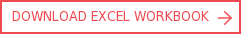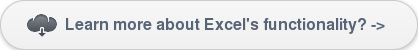View More Content
SHARE

# Use LOOKUP to replace VLOOKUP and HLOOKUP functions in ExcelCategoriesTutorialsOne of the most useful functions available in Excel is the LOOKUP function. This allows you to take any value entered, find it in a data range, then return a value or information from that same data range without having to scroll through a list.## Efficient use of LOOKUP functions in Excel

### LOOKUP

The most commonly used LOOKUP functions in Excel are VLOOKUP and HLOOKUP. VLOOKUP allows you to search a data range that is set up vertically. HLOOKUP is the exact same function, but looks up data that has been formatted by rows instead of columns.

LOOKUP and related functions are commonly used for business analytics in Excel as a way of slicing and dicing data for analysis. We also cover this topic in our Excel training course: The Excel Diploma.

### VLOOKUP and HLOOKUP

However, VLOOKUP and HLOOKUP functions have certain drawbacks that could lead to potential errors, especially for users who are often unfamiliar with the spreadsheet or financial model. These issues will be discussed in this tutorial, together with a simple LOOKUP function which could be used to replace the VLOOKUP/HLOOKUP in the model.

### INDEX and MATCH

In certain cases, especially when there is an unsorted range, a combination of INDEX and MATCH functions could be a more robust solution than the LOOKUP functions. Our downloadable workbook illustrates these concepts – see links at the top or bottom of this page.

INDEX(MATCH) can be a more powerful solution in financial models than the traditional VLOOKUP/HLOOKUP, and can be used to increase the robustness of models in more advanced situations, such as our LBO financial modelling courses and advanced project finance modelling courses.

## Detailed examples of VLOOKUP, HLOOKUP, LOOKUP and INDEX(MATCH)

### VLOOKUP

VLOOKUP searches for a value in the leftmost column of a data range, and then returns a value in the same row from a column you specify in the range. VLOOKUP is used instead of HLOOKUP when your comparison values are located in a column to the left of the data you want to find.

The syntax for VLOOKUP is VLOOKUP (lookup_value, table_array, col_index_num, range_lookup)

‘range_lookup’: If TRUE (or omitted), an approximate match is returned (i.e., if an exact match is not found, the next largest value that is less than ‘lookup_value’ is returned). If FALSE, VLOOKUP will find an exact match.

As an example, sales forecast data is shown in screenshot 1. The first column in the data table is the country; the next few columns includes respective information; and the remaining columns are for the periodic sales forecast data for each country.Screenshot 1: Example of the VLOOKUP function in Excel

### EXAMPLE: Retrieve the periodic sales forecast data for Country "D".

VLOOKUP formula is used to solve as shown in the screenshot. The “col_index_num” for sales forecast in period Mar-09 would be “5”, for period Jun-09 would be “6” and so on. Please note that the “FALSE” is omitted from the “range_lookup” in this example because the data in the first column is sorted (A to J).

### HLOOKUP

HLOOKUP performs the same function as VLOOKUP, but looks up data that has been formatted by rows. HLOOKUP searches for a value in the top row of a table (or an array of values), and then returns a value in the same column from a row you specify in the table or array.

The syntax for HLOOKUP is HLOOKUP (lookup_value, table_array, row_index_num, range_lookup)

The example in the workbook formats the sales forecast data in the VLOOKUP example by rows instead of columns. It demonstrates how the HLOOKUP function is used to look up the periodic sales forecast for the selected country.## PROBLEMS WITH VLOOKUP/HLOOKUP

Use caution if any columns or rows are inserted, moved or deleted:

• If any columns or rows are inserted, moved or deleted within the range, the VLOOKUP and HLOOKUP will return the position of the new column or row in the lookup column/row index number.
• For instance, if a column is inserted between column D and E in screenshot 1, then the user must remember to change the ‘col_index_num’ for sales forecast in period Mar-09 from ‘5’ to ‘6’

VLOOKUP could only search for a value in the first leftmost column of a data range. Similarly, HLOOKUP could only search for a value in the top row of the range:

• When the key field is to the right of the range you want to retrieve, VLOOKUP will not work.
• Using the above example, if you want to retrieve the major client and the country for Priority 1 sales, then VLOOKUP can’t be used, unless the priority column is temporarily moved to the first column in the range.

Values in the first column/row of the data range need to be sorted if range_lookup is ‘TRUE’ (or omitted), unless the range_lookup is ‘FALSE’:

• This could lead to potential errors, especially when the user is not familiar with the function

## HOW TO REPLACE VLOOKUP/HLOOKUP WITH LOOKUP

The VLOOKUP/HLOOKUP functions are often used before a LOOKUP function. However, the LOOKUP function is far cleaner and more transparent, as it only requires two ranges and a LOOKUP value. The syntax for LOOKUP is LOOKUP (lookup_value, lookup_vector, result_vector).Screenshot 2: Example of replacing VLOOKUP with LOOKUP in Excel

Please refer to the accompanying workbook on other examples of using the simple LOOKUP function instead of VLOOKUP/HLOOKUP.

## THE USE OF INDEX(MATCH)

As described above, VLOOKUP and HLOOKUP functions have certain drawbacks that could lead to potential errors, especially for users unfamiliar with the model. Although the simple LOOKUP function could sometimes be used to replace VLOOKUP/HLOOKUP, it is limited to a single row or column to hold results. In some cases, using a combination of INDEX(MATCH) functions may be the only solution, as they are more robust and versatile than VLOOKUP/HLOOKUP.

• INDEX returns the value based on the specific row and column number indexes: INDEX (array, row_num, column_num)
• MATCH returns the position of the matched item in a one-dimensional list: MATCH (lookup_value, lookup_array, match_type) ‘match_type’: If match_type is 0, MATCH finds the first value that is exactly equal to lookup_value (e.g., retrieve the sales forecast for country B for the period ending 30-Jun-09!)Screenshot 3: Example of how INDEX(MATCH) can be used to replace VLOOKUP and LOOKUP

The steps to solve the example using INDEX(MATCH) are:

• Find the ‘row_num’ for country B using the MATCH function – the solution is row # ‘3’
• Find the ‘column_num’ for the period ending Jun-09 using the MATCH function – the solution is column # ‘2’
• The sales forecast can then be solved using the INDEX function, using the ‘row_num’ and ‘column_num’ solved using the MATCH functions aboveby Rickard Wärnelid

Rickard's passion for financial modelling is built on specialist roles in the highly quantitative fields of derivatives and project finance, a career path complemented by an academic grounding in engineering physics. Born in Sweden and with global consulting and leadership experience, Rickard is an internationally recognised authority, speaker and thought-leader on the organisational benefits of best practice financial modelling.

view all